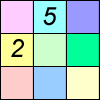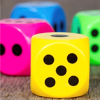Being Resourceful - NumberDozens

Age 7 to 14 Challenge Level:

Do you know a quick way to check if a number is a multiple of two? How about three, four or six?Funny Factorisation

Age 11 to 14 Challenge Level:

Using the digits 1 to 9, the number 4396 can be written as the product of two numbers. Can you find the factors?Where Can We Visit?

Age 11 to 14 Challenge Level:

Charlie and Abi put a counter on 42. They wondered if they could visit all the other numbers on their 1-100 board, moving the counter using just these two operations: x2 and -5. What do you think?Two and Two

Age 11 to 14 Challenge Level:

How many solutions can you find to this sum? Each of the different letters stands for a different number.Stars

Age 11 to 14 Challenge Level:

Can you find a relationship between the number of dots on the circle and the number of steps that will ensure that all points are hit?Product Sudoku

Age 11 to 14 Challenge Level:

The clues for this Sudoku are the product of the numbers in adjacent squares.A Chance to Win?

Age 11 to 14 Challenge Level:

Imagine you were given the chance to win some money... and imagine you had nothing to lose...Difference Sudoku

Age 14 to 16 Challenge Level:

Use the differences to find the solution to this Sudoku.The Remainders Game

Age 7 to 14 Challenge Level:

Play this game and see if you can figure out the computer's chosen number.Nice or Nasty

Age 7 to 14 Challenge Level:

There are nasty versions of this dice game but we'll start with the nice ones...Dicey Operations

Age 11 to 14 Challenge Level:

Who said that adding, subtracting, multiplying and dividing couldn't be fun?How Much Can We Spend?

Age 11 to 14 Challenge Level:

A country has decided to have just two different coins, 3z and 5z coins. Which totals can be made? Is there a largest total that cannot be made? How do you know?Missing Multipliers

Age 7 to 14 Challenge Level:

What is the smallest number of answers you need to reveal in order to work out the missing headers?Cryptarithms

Age 11 to 14 Challenge Level:

Can you crack these cryptarithms?